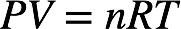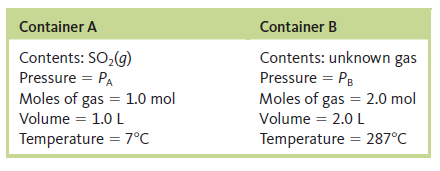# Problem: Consider two separate gas containers at the following conditions:How is the pressure in container B related to the pressure in container A?

###### FREE Expert Solution

Recall: The ideal gas law is as follows:The pressure, volume, temperature and moles of a gas are related to the universal gas constant:This means for two different conditions:Let's designate gas A as SO2 and gas B as the unknown. The identity of the unknown gas is unimportant as long as we know the moles of gas present. Our given values are:
Pa = PA                                                Pb = PB
Va = 1.0 L                                            Vb = 2.0 L
Ta = 7˚C + 273 K = 280 K                  Tb = 287˚C + 273 K = 560 K
na = 1.0 mol                                        nb = 2.0 mol

98% (270 ratings)###### Problem Details

Consider two separate gas containers at the following conditions:How is the pressure in container B related to the pressure in container A?